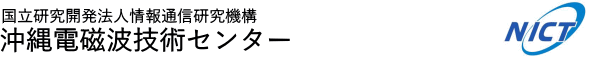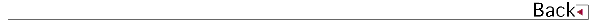# 2009\$BG/EY(B (2009.04\$B!A(B2010.03)

## \$B8&5fO@J8(B

• Camilo Ramos, Michael C. Kelley, Frank T. Djuth, Keith M. Groves, \$BB<;3(B \$BBY7<(B, \$B@nB<(B \$B@?<#(B, Denise Thorsen, Multifrequency observations of Polar Mesosphere Summer Echoes using Alaskan radar facilities: Comparisons and scattering calculations, RADIO SCIENCE, Vol.44, doi:10.1029/2008RS004102, 2009.
• Tetsuya Tagawa, \$B2VEZ(B \$B90(B, Shuji Shimizu, Riko Oki, Improved Correction of Beam Mismatch of the Precipitation Radar After Orbit Boost of the TRMM Satellite, IEEE TRANSACTIONS ON GEOSCIENCE AND REMOTE SENSING, Vol.47, No.10, P.3469-3479, 2009.
• Nanan Balan, \$B1v@nOBIW(B, \$BBgDMM:0l(B, \$B5FCS?r(B, D. Vijaya Lekshmi, \$B@nB<(B \$B@?<#(B, \$B;3K\1R(B, G. J. Bailey, A physical mechanism of positive ionospheric storms at low and 2 mid latitudes, JOURNAL OF GEOPHYSICAL RESEARCH, Vol.115, A02304, doi:10.1029/2009JA014515, 2010.

## \$B>.O@J8(B

• \$B4X_7(B \$B?.Li(B, \$B@nB<(B \$B@?<#(B, \$BB<;3(B \$BBY7<(B, \$BET;TBg5\$\$N9bL)EYN)BN4QB,\$N\$?\$a\$N9b%Q%k%905=L%&%#%s%I%W%m%U%!%\$%i\$N3+H/(B, \$BEE;R>pJsDL?.3X2qO@J8;o(BB, Vol.J93-B, No.1, P.127 -131, 2010.
• \$B4X_7(B \$B?.Li(B, \$B@nB<(B \$B@?<#(B, \$B1J0f(B \$B@6Fs(B, \$B:4C](B \$B@?(B, \$BCf@n(B \$B>!9-(B, 400MHz\$BBS%&%#%s%I%W%m%U%!%\$%i\$rMQ\$\$\$?1+E)N37BJ,I[\$N?dDj(B, \$BEE;R>pJsDL?.3X2q(B \$BO@J8;o(BB, Vol.J93-B, No.3, P.564-568, 2010.

## \$B<}O?O@J8(B

• \$B;0>e(B \$B:L(B, \$B8EK\(B \$B=_0l(B, \$BDEED(B \$BIRN4(B, \$B:4F#(B \$B?82p(B, \$B1J0f(B \$B@6Fs(B, \$BB<;3(B \$BBY7<(B, \$B@nH*(B \$BBsLp(B, Meso-gamma-scale convective systems observed by a 443 MHz wind-profiling radar with RASS in the Okinawa subtropical regions, MST12 Workshop, No.T5.15, 2009.
• \$B=P@\$(B \$B\$f\$+\$j(B, \$B:4C](B \$B@?(B, \$B:4F#(B \$B?82p(B, \$B9b66(B \$BD*9((B, \$B2VEZ(B \$B90(B, \$BCf@n(B \$B>!9-(B, \$B0f8}(B \$B=SIW(B, POLARIMETRIC RADAR OBSERVATION OF THE EYEWALL OF TYPHOON MAN-YI, 34th Conference on Radar Meteorology (American Meteorological Society), P13-23, 2009.
• \$B@nB<(B \$B@?<#(B, \$B2VEZ(B \$B90(B, \$B?yC+(B \$BLPIW(B, \$BCf@n(B \$B>!9-(B, \$B2-FlJPGH9_1+%l!<%@\$K\$h\$k2~NI7?%P%\$%9%?%F%#%C%/9_1+4QB,

## \$B0lHL8}F,H/I=(B

• \$B7*86(B \$B59;R(B, \$BLn_7(B \$B8gFA(B, \$BBg;3!!?-0lO:(B, \$BF#0f(B \$BNI0l(B, \$BB<;3(B \$BBY7<(B, \$B@nB<(B \$B@?<#(B, \$B%H%m%`%=(BMF\$B%l!<%@!<%0%k!<%W(B, MF\$B%l!<%@!<\$G4QB,\$5\$l\$?6K0hCf4V7w\$K\$*\$1\$kBg5\$D,<.GH(B, \$BF|K\CO5eOG@12J3XO"9g(B2009\$BG/Bg2q(B, No.E117-P010, 2009.
• \$B:dLn0f(B \$BOBBe(B, \$BB<;3(B \$BBY7<(B, Richard L. Collins, \$B?eC+(B \$B9LJ?(B, \$B@nB<(B \$B@?<#(B, \$B%"%i%9%+>e6u\$K\$*\$1\$kE_5(CfAXBg5\$JQF0\$ND94|2r@O(B, \$BF|K\CO5eOG@12J3XO"9g(B2009\$BG/Bg2q(B No.E117-P007, 2009.
• \$B:4F#(B \$B?82p(B, \$B1J0f(B \$B@6Fs(B, \$BB<;3(B \$BBY7<(B, \$B0f8}(B \$B=SIW(B, \$B7'C+(B \$BGn(B, \$B8EK\(B \$B=_0l(B, \$BDEED(B \$BIRN4(B, 400MHz\$BBS(BWPR/RASS\$B\$K\$h\$kE_5(2-Fl\$NBg5\$6-3&AX\$N4QB,(B, \$BF|K\5\$>]3X2q(B \$B=U4|Bg2q(B, No.C205, 2009.
• \$B@nH*(B \$BBsLp(B, \$B;0>e(B \$B:L(B, \$B8EK\(B \$B=_0l(B, \$B:4F#(B \$B?82p(B, \$B1J0f(B \$B@6Fs(B, RASS\$B4QB,%G!<%?\$rMQ\$\$\$?2-FlK\Eg\$K\$*\$1\$k>.5,LO9_?eBS\$NF12=!&M=Js]3X2q(B \$B=U4|Bg2q(B, No.B301, 2009.
• \$B=P@\$(B \$B\$f\$+\$j(B, \$B:4C](B \$B@?(B, \$B:4F#(B \$B?82p(B, \$B9b66(B \$BD*9((B, \$B2VEZ(B \$B90(B, \$BCf@n(B \$B>!9-(B, \$B0f8}(B \$B=SIW(B, \$BBfIw(B0704\$B9f\$K\$*\$1\$k(BCOBRA \$BJPGH%Q%i%a!<%?\$H9_?eN3;RJ,I[FC@-(B, \$BF|K\5\$>]3X2q(B \$B=U4|Bg2q(B, No.D409, 2009.
• \$B:4C](B \$B@?(B, \$B@nB<(B \$B@?<#(B, \$B=P@\$\$f\$+\$j(B, \$BCf@n(B \$B>!9-(B, \$B0f8}(B \$B=SIW(B, Ocean surface backscattering at extremely low grazing angles observed by C-band polarimetric doppler weather radar, 2009 IEEE International Geoscience & Remote Sensing Symposium, No.WE1.O3.5, 2009.
• \$B7*86(B \$B59;R(B, \$BLn_7(B \$B8gFA(B, \$BBg;3(B \$B?-0lO:(B, \$BF#0f(B \$BNI0l(B, \$B@nB<(B \$B@?<#(B, \$BB<;3(B \$BBY7<(B, Chirs Hall, Characteristics of atmospheric tidal wave in the polar mesosphere observed with two MF radars at Tromso and Poker Flat over 10 years, EISCAT Workshop 2009, 2009.
• \$BCf@n(B \$B>!9-(B, \$B2VEZ(B \$B90(B, \$B@nB<(B \$B@?<#(B, \$B0f8}(B \$B=SIW(B, Rain Attenuation Estimation for the GPM/DPR Algorithm by use of the C-band Polarimetric Radar and the 400-MHz Wind Profiler, AOGS2009 (Asia Oceania Geosciences Society), 2009.
• \$B@nB<(B \$B@?<#(B, \$BCf@n(B \$B>!9-(B, \$B4X_7(B \$B?.Li(B, \$B?yC+(B \$BLPIW(B, \$B1J0f(B \$B@6Fs(B, \$B2-Fl(B400MHz\$BBS%&%#%s%I%W%m%U%!%\$%i\$K\$h\$k1+E)N37BJ,I[?dDj(B, \$BCO5eEE<'5\$!&CO5eOG@17w3X2q(B \$BAm2q5Z\$S9V1i2q(B (SGEPSS), 2009.
• \$B:dLn0f(B \$BOBBe(B, \$BB<;3(B \$BBY7<(B, Richard L. Collins, \$B?eC+(B \$B9LJ?(B, \$B@nB<(B \$B@?<#(B, \$B%"%i%9%+>e6u\$K\$*\$1\$kE_5(CfAXBg5\$JQF0\$ND94|2r@O#2(B, \$BCO5eEE<'5\$!&CO5eOG@17w3X2q(B \$BAm2q5Z\$S9V1i2q(B (SGEPSS), No.B005-10, 2009.
• \$BFsC+(B \$B?rBg(B, \$B;0Bp(B \$BATAo(B, \$B@P:d(B \$B7=8c(B, \$B0286(B \$BM\$
• \$B7*86(B \$B59;R(B, \$BLn_7(B \$B8gFA(B, \$BBg;3(B \$B?-0lO:(B, \$BF#0f(B \$BNI0l(B, \$B@nB<(B \$B@?<#(B, \$BB<;3(B \$BBY7<(B, \$B%H%m%`%=#M#F%l!<%@!<%0%k!<%W(B, \$B%H%m%`%=!&%]!<%+%U%i%C%H(BMF\$B%l!<%@!<\$ND94|4V4QB,%G!<%?\$K\$h\$k6K0hCf4V7w\$NBg5\$D,<.GH\$NE}7WE*2r@O(B, \$BCO5eEE<'5\$!&CO5eOG@17w3X2q(B \$BAm2q5Z\$S9V1i2q(B (SGEPSS), No.B005-11, 2009.
• \$BCf@n(B \$B>!9-(B, \$B@nB<(B \$B@?<#(B, \$B4X_7(B \$B?.Li(B, \$B2VEZ(B \$B90(B, \$B0f8}(B \$B=SIW(B, Vertical Rain Attenuation Characteristics of Ka- and Ku-band observed by the 400-MHz Wind Profiler, 34th AMS Conference on Radar Meteorology, No.P4.3, 2009.
• \$BCf@n(B \$B>!9-(B, \$B2VEZ(B \$B90(B, \$B0f8}(B \$B=SIW(B, \$B2-(B \$BM};R(B, \$B@6?e(B \$B<};J(B, \$BCfB<(B \$B7r<#(B, Ground Validation and Calibration for GPM/DPR, International Symposium on Radar and Modeling Studies of the Atmosphere, No.I16, 2009.
• \$B@nB<(B \$B@?<#(B, \$BCf@n(B \$B>!9-(B, \$B2VEZ(B \$B90(B, \$B=P@\$(B \$B\$f\$+\$j(B, \$B:4C](B \$B@?(B, \$B:4F#(B \$B?82p(B, \$B9b66(B \$BD*9((B, \$B0f8}(B \$B=SIW(B, Research and development of the COBRA at NICT, International Symposium on Radar and Modeling Studies of the Atmosphere, 2009.
• \$B7*86(B \$B59;R(B, \$BLn_7(B \$B8gFA(B, \$BBg;3(B \$B?-0lO:(B, \$BF#0f(B \$BNI0l(B, \$B@nB<(B \$B@?<#(B, \$BB<;3(B \$BBY7<(B, Chirs Hall, \$B%H%m%`%=!&%]!<%+%U%i%C%H(BMF\$B%l!<%@!<\$ND94|4V4QB,\$+\$iF@\$i\$l\$?6K0hCf4V7w\$NBg5\$D,<.GH\$NFCD'(B, \$BBh(B33\$B2s6K0hCh6u7w%7%s%]%8%&%`(B, 2009.
• \$BOBED(B \$B2ZBe(B, \$BNkLZ(B \$B8-;N(B, \$BCfKL(B \$B1Q0l(B, \$B;38}(B \$B90@?(B, \$BBg@P(B \$BE/(B, \$BDZLZ(B \$BOB5W(B, \$B=P@\$(B \$B\$f\$+\$j(B, \$BBgEl(B \$BCiJ](B, \$BCf@n(B \$B>!9-(B, \$B@nB<(B \$B@?<#(B, \$B9b66(B \$BR#(B, \$B2-FlJPGH9_1+%l!<%@!<(BCOBRA\$B\$H%S%G%*%>%s%G\$K\$h\$kF14|4QB,(B-2009\$BG/%S%G%*%>%s%G4QB,\$N35MW(B-, \$BF|K\5\$>]3X2q(B \$B=)5(Bg2q(B, No.P120, P.352-352, 2009.
• \$B@nB<(B \$B@?<#(B, \$B2VEZ(B \$B90(B, \$B?yC+(B \$BLPIW(B, \$BCf@n(B \$B>!9-(B, \$B%G%8%?%k%S!<%`%U%)!<%_%s%0\$rMxMQ\$7\$?2~NI7?%P%\$%9%?%F%#%C%/9_1+%l!<%@]3X2q(B \$B=)5(Bg2q(B, 2009.
• \$B:4F#(B \$B?82p(B, \$B8EK\(B \$B=_0l(B, \$B1J0f(B \$B@6Fs(B, \$B@nB<(B \$B@?<#(B, \$B2VEZ(B \$B90(B, \$BCf@n(B \$B>!9-(B, \$BB<;3(B \$BBY7<(B, \$BDEED(B \$BIRN4(B, 400MHz\$BBS(BWPR/RASS\$B\$K\$h\$kG_1+A0@~BS\$N9_?e2aDx\$H29EY>l\$N4QB,(B, \$BF|K\5\$>]3X2q(B \$B=)4|Bg2q(B, No.D102, 2009.
• \$B8EK\(B \$B=_0l(B, \$BDEED(B \$BIRN4(B, \$B:4F#(B \$B?82p(B, \$B1J0f(B \$B@6Fs(B, \$BB<;3(B \$BBY7<(B, \$B@nH*(B \$BBsLp(B, \$B:XF#(B \$BOBM:(B, 400MHz\$BBS(BWPR\$B!&(BRASS\$B\$K\$h\$k0!G.BS0h5\$29O"B34QB,\$N@:EY8!>Z(B, \$BF|K\5\$>]3X2q(B \$B=)4|Bg2q(B, No.P317, 2009.
• \$BCf@n(B \$B>!9-(B, \$B2VEZ(B \$B90(B, \$B9b66(B \$BD*9((B, \$B0f8}(B \$B=SIW(B, \$B>.Eh@590(B, \$B8E@n6U;J(B, \$BI4B+BY=S(B, \$B@P@Z9'G7(B, \$B;01:7r;K(B, \$B@6?e<};J(B, \$B1|B<e9;@5J}K!\$N8!F\$(B, \$BF|K\5\$>]3X2q(B \$B=)5(Bg2q(B, No.P314, 2009.
• \$B5WJ]EDBs;V(B, \$B1J8M5W4n(B, \$B@DM|OB@5(B, \$B66K\L@90(B, \$B0f8}(B \$B=SIW(B, \$B2VEZ(B \$B90(B, \$B@6?e<};J(B, \$B5HEDD>J8(B, \$B2-M};R(B, \$B1R@1Ek:\9_?e%l!<%@%7%_%e%l!<%?\$K\$h\$k#J#M#A(B?\$B#N#H#M1@HyJ*M}2aDx\$N8!>Z(B, \$BF|K\5\$>]3X2q(B \$B=)5(Bg2q(B, No.D212, P.296-296, 2009.
• \$B?yC+(B \$BLPIW(B, \$B@nB<(B \$B@?<#(B, \$B2VEZ(B \$B90(B, \$BCf@n(B \$B>!9-(B, \$B2-FlJPGH9_1+%l!<%@(BCOBRA\$B\$NAw?.JPGH9;@5:n6H\$N>JNO2=\$H9b@:EY2=(B, \$BF|K\5\$>]3X2q(B \$B=)5(Bg2q(B, Vol.96, No.D359, P.321-321, 2009.
• \$B7*86(B \$B59;R(B, \$BLn_7(B \$B8gFA(B, \$BBg;3(B \$B?-0lO:(B, \$BF#0f(B \$BNI0l(B, \$B@nB<(B \$B@?<#(B, \$BB<;3(B \$BBY7<(B, Chirs Hall, \$B%H%m%`%=!&%]!<%+%U%i%C%H(BMF\$B%l!<%@!<\$G4QB,\$5\$l\$?6K0hCf4V7w\$K\$*\$1\$kBg5\$D,<.GH\$N5(@a?d0\(B, \$BCf4V7w!&G.7w!&EEN%7w(B(MTI)\$B8&5f2q(B, 2009.
• \$B2VEZ(B \$B90(B, GPM(\$BA45e9_?e4QB,7W2h(B)\$Be9;@5J}K!\$N8!F\$(B, \$BCfB<8&(BNICT\$B@iMUBg9gF1%;%_%J!
• \$B:4F#(B \$B?82p(B, \$B8EK\(B \$B=_0l(B, \$B1J0f(B \$B@6Fs(B, \$B@nB<(B \$B@?<#(B, \$B2VEZ(B \$B90(B, \$BCf@n(B \$B>!9-(B, \$BB<;3(B \$BBY7<(B, \$BDEED(B \$BIRN4(B, Rainfall and temperature profiles in a Baiu frontal zone observed by a 400 MHz band wind profiling radar with RASS", \$BCfB<8&(BNICT\$B@iMUBg9gF1%;%_%J!
• \$B@nB<(B \$B@?<#(B, \$B2VEZ(B \$B90(B, \$B?yC+(B \$BLPIW(B, \$BCf@n(B \$B>!9-(B, COBRA\$B2~NI7?%P%\$%9%?%F%#%C%/\$K\$h\$k(B2\$Bl?dDj\$N;n\$_(B, COBRA\$B8&5f=82q(B, 2009.
• \$B:4F#(B \$B?82p(B, \$B8EK\(B \$B=_0l(B, \$B1J0f(B \$B@6Fs(B, \$B@nB<(B \$B@?<#(B, \$B2VEZ(B \$B90(B, \$BCf@n(B \$B>!9-(B, \$BB<;3(B \$BBY7<(B, \$BDEED(B \$BIRN4(B, 400MHz\$BBS(BWPR/RASS\$B\$K\$h\$k2-FlG_1+4|\$NAX>u@-9_?e\$H29EY>l\$N4QB,(B, \$B@hC
• \$B@nB<(B \$B@?<#(B, \$B?yC+(B \$BLPIW(B, \$B2VEZ(B \$B90(B, \$BCf@n(B \$B>!9-(B, \$B2~NI7?%P%\$%9%?%F%#%C%/9_1+%l!<%@
• \$BB<;3(B \$BBY7<(B, \$B4d0f(B \$B9(FA(B, \$B@nB<(B \$B@?<#(B, \$B4X_7(B \$B?.Li(B, \$B>o>>(B \$BE8=<(B, \$B@P0f(B \$B>;7{(B, \$B?eC+(B \$B9LJ?(B, NICT\$B\$K\$h\$k6-3&AX%j%b!<%H%;%s%7%s%04QB,%W%m%8%'%/%H\$N?JD=\$K\$D\$\$\$F(B, \$BF|K\CO5eOG@12J3XO"9g(B2009\$BG/Bg2q(B, 2009.
• \$BB<;3(B \$BBY7<(B, \$B4d0f(B \$B9(FA(B, \$B4X_7(B \$B?.Li(B, \$B@P0f(B \$B>;7{(B, \$B?eC+(B \$B9LJ?(B, \$B>.ED(B \$BN=;R(B, \$B@nB<(B \$B@?<#(B, \$B%l!<%@!.5,LO>qMp\$N4QB,(B, \$BBh(B126\$B2s(BSGEPSS\$B9V1i2q(B, 2009.
• \$B>.@n(B \$BCiI'(B, \$BB<;3(B \$BBY7<(B, \$B@nB<(B \$B@?<#(B, \$BCUFb(BVHF\$B%l!<%@!<\$H(BMF\$B%l!<%@!<\$GF1;~4QB,\$5\$l\$?2F5(Cf4V7w%(%3!<(B, \$BBh(B126\$B2s(B SGEPSS\$BAm2q(B, 2009.
• \$BB<;3(B \$BBY7<(B, \$B4d0f(B \$B9(FA(B, \$B4X_7(B \$B?.Li(B, \$B>.ED(B \$BN=;R(B, \$B?eC+(B \$B9LJ?(B, \$B@P0f(B \$B>;7{(B, \$B@nB<(B \$B@?<#(B, \$BBgLn(B \$BM50l(B, \$BBg5\$6-3&AX\$N%l!<%@!
• \$BB<;3(B \$BBY7<(B, \$B@nB<(B \$B@?<#(B, \$BDE@n(B \$BBnLi(B, \$B@P0f(B \$B
• \$B>.ED(B \$BN=;R(B, \$B4d0f(B \$B9(FA(B, \$BBgLn(B \$BM50l(B, \$B@nB<(B \$B@?<#(B, \$B4X_7(B \$B?.Li(B, \$B@P0f(B \$B>;7{(B, \$B?eC+(B \$B9LJ?(B, \$BB<;3(B \$BBY7<(B, \$BEl5~ET>.6b0f;T>e6u\$N6-3&AX9bEYF|JQ2=\$N?dDj(B, \$BF|K\5\$>]3X2q(B \$B=U5(Bg2q(B, 2010.
• \$B9b66Bg2p(B, \$B?9K\>

## 1990\$B!A(B1991\$B!d(B1992\$B!d(B1993\$B!d(B1994\$B!d(B1995\$B!d(B1996\$B!d(B1997\$B!d(B1998\$B!d(B 1999\$B!d(B2000\$B!d(B2001\$B!d(B2002\$B!d(B2003\$B!d(B2004\$B!d(B2005\$B!d(B2006\$B!d(B2007\$B!d(B 2008\$B!d(B2009\$B!d(B2010\$B!d(B2011\$B!d(B2012\$B!d(B2013\$B!d(B2014\$B!d(B2015\$B!d(B2016\$B!d(B2017